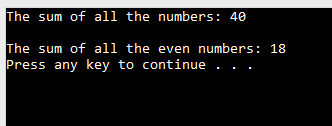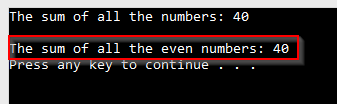The Liskov Substitution Principle (LSP) states that child class objects should be able to replace parent class objects without compromising application integrity. What this means essentially, is that we should put an effort to create such derived class objects which can replace objects of the base class without modifying its behavior. If we don’t, our application might end up being broken.

Does this make sense to you?

To make things clear, we are going to use a simple „Sum Calculator“ example, which will help us to understand how to implement the LSP better.

To download the source code for this project, check out the Liskov Substitution Principle Project Source Code.

To read about other SOLID principles, check out our SOLID Principles page.

## Initial Project

In this example, we are going to have an array of numbers and a base functionality to sum all the numbers from that array. But let’s say we need to sum just even or just odd numbers.

How would we implement that? Let’s see one way to do it:

```public class SumCalculator
{

public SumCalculator(int[] numbers)
{
_numbers = numbers;
}

public int Calculate() => _numbers.Sum();
}
```
```public class EvenNumbersSumCalculator: SumCalculator
{
:base(numbers)
{
}

public new int Calculate() => _numbers.Where(x => x % 2 == 0).Sum();
}
```

Now if we test this solution, whether we calculate the sum of all the numbers or the sum of just even numbers, we are going to get the correct result for sure:

```class Program
{
static void Main(string[] args)
{
var numbers = new int[] { 5, 7, 9, 8, 1, 6, 4 };

SumCalculator sum = new SumCalculator(numbers);
Console.WriteLine(\$"The sum of all the numbers: {sum.Calculate()}");

Console.WriteLine();

Console.WriteLine(\$"The sum of all the even numbers: {evenSum.Calculate()}");
}
}
```

The result is:## Creating a Better Solution

As we can see, this is working just fine. But what is wrong with this solution then?

Why are we trying to fix it?

Well, as we all know, if a child class inherits from a parent class, then the child class is a parent class. Having that in mind, we should be able to store a reference to an `EvenNumbersSumCalculator` as a `SumCalculator` variable and nothing should change. So, let’s check that out:

```SumCalculator evenSum = new EvenNumbersSumCalculator(numbers);
Console.WriteLine(\$"The sum of all the even numbers: {evenSum.Calculate()}");
```As we can see, we are not getting the expected result because our variable `evenSum` is of type `SumCalculator` which is a higher order class (a base class). This means that the `Count` method from the `SumCalculator` will be executed. So, this is not right, obviously, because our child class is not behaving as a substitute for the parent class.

Luckily, the solution is quite simple. All we have to do is to implement small modifications to both of our classes:

```public class SumCalculator
{

public SumCalculator(int[] numbers)
{
_numbers = numbers;
}

public virtual int Calculate() => _numbers.Sum();
}
```

```public class EvenNumbersSumCalculator: SumCalculator
{
:base(numbers)
{
}

public override int Calculate() => _numbers.Where(x => x % 2 == 0).Sum();
}
```

As a result, when we start our solution, everything works as expected and the sum of even numbers is 18 again.

So, let’s explain this behavior.  If we have a child object reference stored in a parent object variable and call the `Calculate` method, the compiler will use the `Calculate` method of the parent class. But right now because the `Calculate` method is defined as „virtual“ and is overridden in the child class, that method in the child class will be used instead.

## Implementing the Liskov Substitution Principle

Still, the behavior of our derived class has changed and it can’t replace the base class. So we need to upgrade this solution by introducing the `Calculator` abstract class:

```public abstract class Calculator
{

public Calculator(int[] numbers)
{
_numbers = numbers;
}

public abstract int Calculate();
}```

Then we have to change our other classes:

```public class SumCalculator : Calculator
{
public SumCalculator(int[] numbers)
:base(numbers)
{
}

public override int Calculate() => _numbers.Sum();
}```
```public class EvenNumbersSumCalculator: Calculator
{
:base(numbers)
{
}

public override int Calculate() => _numbers.Where(x => x % 2 == 0).Sum();
}```

Excellent. Now we can start making calls towards these classes:

``` class Program
{
static void Main(string[] args)
{
var numbers = new int[] { 5, 7, 9, 8, 1, 6, 4 };

Calculator sum = new SumCalculator(numbers);
Console.WriteLine(\$"The sum of all the numbers: {sum.Calculate()}");

Console.WriteLine();

Console.WriteLine(\$"The sum of all the even numbers: {evenSum.Calculate()}");
}
}```

We will again have the same result, 40 for all the numbers and 18 for the even numbers. But now, we can see that we can store any subclass reference into a base class variable and the behavior won’t change which is the goal of LSP.

## What We Gain By Implementing the LSP

By implementing the LSP, we are keeping our functionality intact and still having our subclasses act as a substitute to a base class.

Also, we encourage the code reusability by implementing the LCP and having better project maintenance as well.

## Conclusion

We can see that implementing the LSP is not that complicated but just the opposite. Most of us probably already implemented this principle many times in our code without knowing its name because in the object-oriented world Polymorphism is quite a big thing.